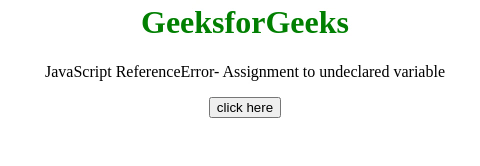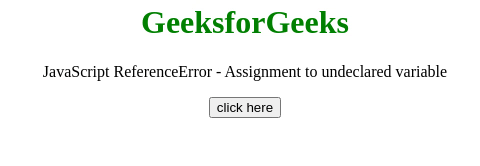# JavaScript ReferenceError – Assignment to undeclared variable

• Last Updated : 24 Jul, 2020

This JavaScript exception Assignment to undeclared variable occurs in strict-mode If the value has been assigned to an undeclared variable.

Message:

Hey geek! The constant emerging technologies in the world of web development always keeps the excitement for this subject through the roof. But before you tackle the big projects, we suggest you start by learning the basics. Kickstart your web development journey by learning JS concepts with our JavaScript Course. Now at it's lowest price ever!

```ReferenceError: assignment to undeclared variable "x" (Firefox)
ReferenceError: "x" is not defined (Chrome)
ReferenceError: Variable undefined in strict mode (Edge)
```

Error Type:

```ReferenceError
```

Cause of the error: Somewhere in the code, there is an assignment without the var, let or const keyword. When a value is assigned to an undeclared variable this error occurs.

Example 1: In this example, the const keyword is used with the variable assignment, So the error has not occurred.

## HTML

 ```<``html``>  ``<``head``>     ``   ``<``body` `style``=``"text-align:center;"``> ``    ``<``h1` `style``=``"color:green;"``>  ``        ``GeeksforGeeks  ``    `` ``     ` `    ``<``p``>``        ``JavaScript ReferenceError-``        ``Assignment to undeclared variable ``    ````    ` `    ``<``button` `onclick` `= ``"Geeks();"``>``        ``click here``    ````    ``<``p` `id``=``"GFG_DOWN"``> ``    ````        ` `    ``<``script``> ``        ``var el_down = document.getElementById("GFG_DOWN");``        ``function GFG() { ``          ``'use strict'; ``           ``const var_1 = "Value assigned without declaration"; ``        ``}``        ``function Geeks() { ``            ``try {``                ``GFG();``                ``el_down.innerHTML = ``                ``"'Assignment to undeclared variable'"+``                ``"error has not occurred";``            ``} catch(e) {``                ``el_down.innerHTML = ``                ``"'Assignment to undeclared variable'"+``                ``"error has occurred";``            ``}``        ``} ``    `` ``   ```

Output:Example 2: In this Example, the var, let or const keyword is not used with the variable assignment, So the error has occurred.

## HTML

 ```<``html``>  ``<``head``>     ``   ``<``body` `style``=``"text-align:center;"``> ``    ``<``h1` `style``=``"color:green;"``>  ``        ``GeeksforGeeks  ``    `` ``     ` `    ``<``p``>``        ``JavaScript ReferenceError -``        ``Assignment to undeclared variable ``    ````    ` `    ``<``button` `onclick` `= ``"Geeks();"``>``        ``click here``    ````    ``<``p` `id``=``"GFG_DOWN"``> ``    ````        ` `    ``<``script``> ``        ``var el_down = document.getElementById("GFG_DOWN");``        ``function GFG() { ``          ``'use strict'; ``          ``var_1 = true; ``        ``}``        ``function Geeks() { ``            ``try {``                ``GFG();``                ``el_down.innerHTML = ``                ``"'Assignment to undeclared variable'"+``                ``"error has not occurred";``            ``} catch(e) {``                ``el_down.innerHTML = ``                ``"'Assignment to undeclared variable'"+``                ``"error has occurred";``            ``}``        ``} ``    `` ``   ```

Output:My Personal Notes arrow_drop_up